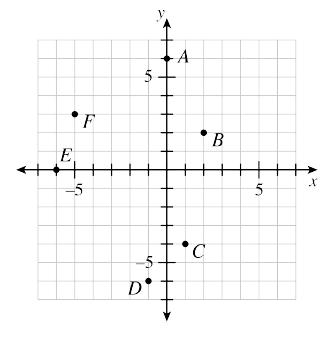### Home > CC1MN > Chapter 8 > Lesson 8.2.5 > Problem8-119

8-119.This problem is a checkpoint for locating points on a number line and on a coordinate graph. It will be referred to as Checkpoint 8.

1. Indicate the approximate location of each number on a number line.

$−2$, $4$, $−1.7$, $\frac { 3 } { 4 }$, $−0.2$$-\frac{10}{3}$$4\frac{1}{5}$, $150\%$

2. What are the coordinates of each named point on the coordinate graph?Check your answers by referring to the Checkpoint 8 materials located at the back of your book.

Ideally, at this point you are comfortable working with these types of problems and can solve them correctly. If you feel that you need more confidence when solving these types of problems, then review the Checkpoint 8 materials and try the practice problems provided. From this point on, you will be expected to do problems like these correctly and with confidence.

Answers and extra practice for the Checkpoint problems are located in the back of your printed textbook or in the Reference Tab of your eBook. If you have an eBook for Core Connections, Course 1, login and then click the following link:  Checkpoint 8: Locating Points on a Number Line and on a Coordinate Graph